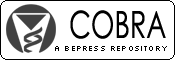## COBRA Preprint Series

#### Abstract

Estimates of minimum sample sizes are calculated in order to test differences in rates of changes over time for longitudinal designs. In this study, body weight of crossbred beef cattle, considering 14 measurements on individuals, taken at birth, weaning (7 months of age) and monthly from 8 to 19 months of age, were analyzed by an usual mixed model for repeated measures. The number of individuals n required to detect significant differences (delta) between any two consecutive measurements on the individual, was obtained by a SAS program considering a t-variate normal distribution (t = 14), sample variance–covariance matrix among the repeated measures, F-distribution with noncentrality parameter, type I error (alpha), power test (1-beta) and minimum correlation between repeated measures. Figures showing the n estimated as function of number of measurements on the individual, alpha (0.01 and 0.05); power of test (0.80 and 0.90); minimum correlation (0, 0.2, 0.4 and 0.6) and delta (1.0 standard deviation, 1.5 standard deviation and 2.0 standard deviation) are presented. Keywords: Longitudinal data, power calculation, repeated measures, sample size estimate.

#### Disciplines

Longitudinal Data Analysis and Time Series

COinS